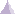# Membrane PropertyMembrane property is a physical property assigned to surface elements. This property can be applied on linear triangle elements (TR3), parabolic triangle elements (TR6), linear quadrangle elements (QD4) and parabolic quadrangle elements (QD8).

A membrane property references a material assigned to the surface part and describes a thickness associated to this surface part. A membrane property is associative to the geometry this property points at.

Associated to this property, elements (linear or parabolic triangle, linear or parabolic quadrangle) have:

• a plane stress state,
• two degrees of freedom per node (both translations in the finite element plane),
• no transversal stiffness,
• longitudinal shearing,
• tension / compression deformation.

The input and output characteristics are:

• Input:

• Material

• Thickness

• Output:

• Stress

• Strain

• Point force vector

• Stress Von Mises
• Elastic energy
• Elastic energy density
• Estimated error

Those characteristics can be expressed at the given positions in the elements and in different axis systems:

 Position Axis System Characteristics Center of element Nodes of element Gauss point Global Local StressStrainPoint force vectorStress Von MisesElastic energyElastic energy densityEstimated error